# Cube Volume & Surface Area Calculator

Length
Volume  =  125in³GENERATE WORK

## Cube Volume - work with steps

Input Data :
Length = 5 in

Objective :
Find the volume of the cube.

Formula :
Volume = a3

Solution :
Volume = 53
Volume = 125 in³

Cube volume and surface area calculator uses side length of a cube, and calculates the surface area and volume of the cube. It is an online Geometry tool requires side length of a cube. Using this calculator, we will find the surface area and volume of the three-dimensional solid bounded by six square faces.
It is necessary to follow the next steps:

1. Enter the side length of a cube in the box. The value must be positive real number or parameter. Note that the length of a segment is always positive;
2. Press the "GENERATE WORK" button to make the computation;
3. Cube surface area and volume calculator will give the surface area and volume of a cube.
Input: A positive real number or parameter as the side length of a cube;
Output: Two positive real numbers or variables as the surface area and volume of a cube and corresponding units after that.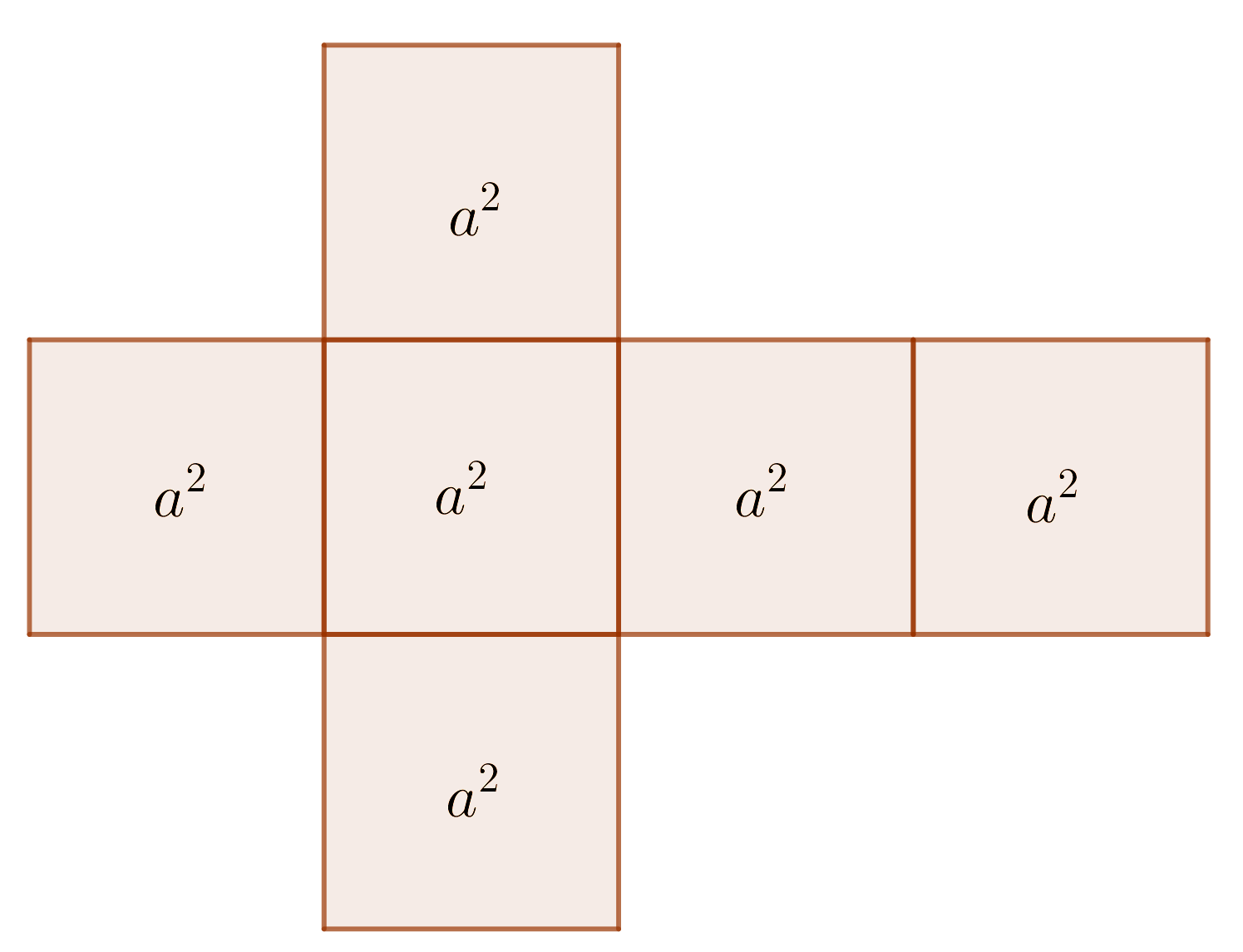Cube Formula :
Surface Area of Cube Formula:
The surface area, $S$, of a cube is determined by the following formula
$$S = 6\times a\times a=6\times a^2$$
where $a$ is the length of the side of the cube.

Volume of Cube Formula:
The volume, $V$, of a cube is determined by the following formula
$$V =\mbox{length}\times\mbox{width}\times\mbox{height}= a\times a\times a=a^3$$
where $a$ is the length of the side of the cube.

## How to Find the Surface Area & Volume of a Cube?

A polyhedron is a three-dimensional geometric object bounded by polygons. These polygons are called its faces.A common side of two adjacent faces of the polyhedron is called an edge or side of the polyhedron. Three or more faces meet at common vertex which is called a vertex of the polyhedron. A polyhedron is a regular polyhedron if all of its faces are regular congruent polygons and all of the edges are congruent. There are five convex regular polyhedron, known as the Platonic solids: tetrahedron, cube, octahedron, dodecahedron, icosahedron.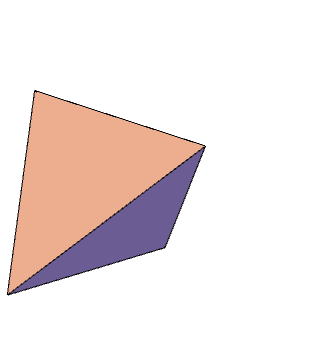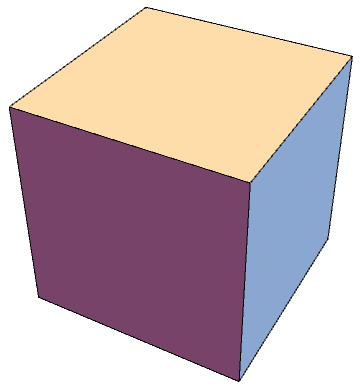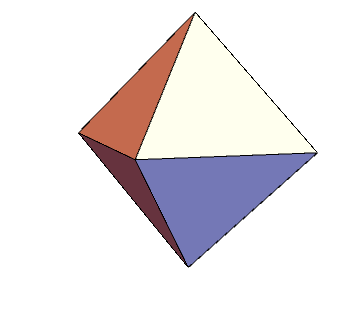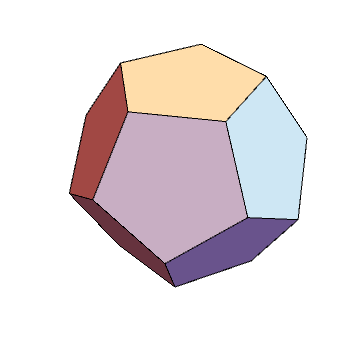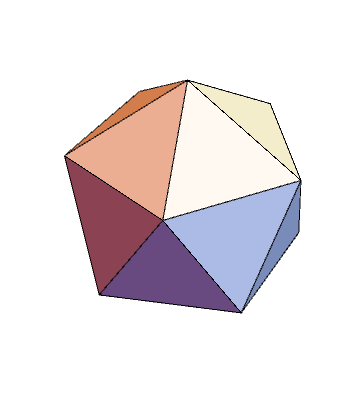Tetrahedron, cube, octahedron, dodecahedron, icosahedron
A prism is a polyhedron with two parallel congruent faces called bases. The other faces are parallelograms. Prisms are usually named by the shape of their bases. A regular prism is a prism with bases of regular polygons. A cube is an example of a regular prism. A cube has $6$ faces, $8$ vertices and $12$ edges and all faces of a cube are squares.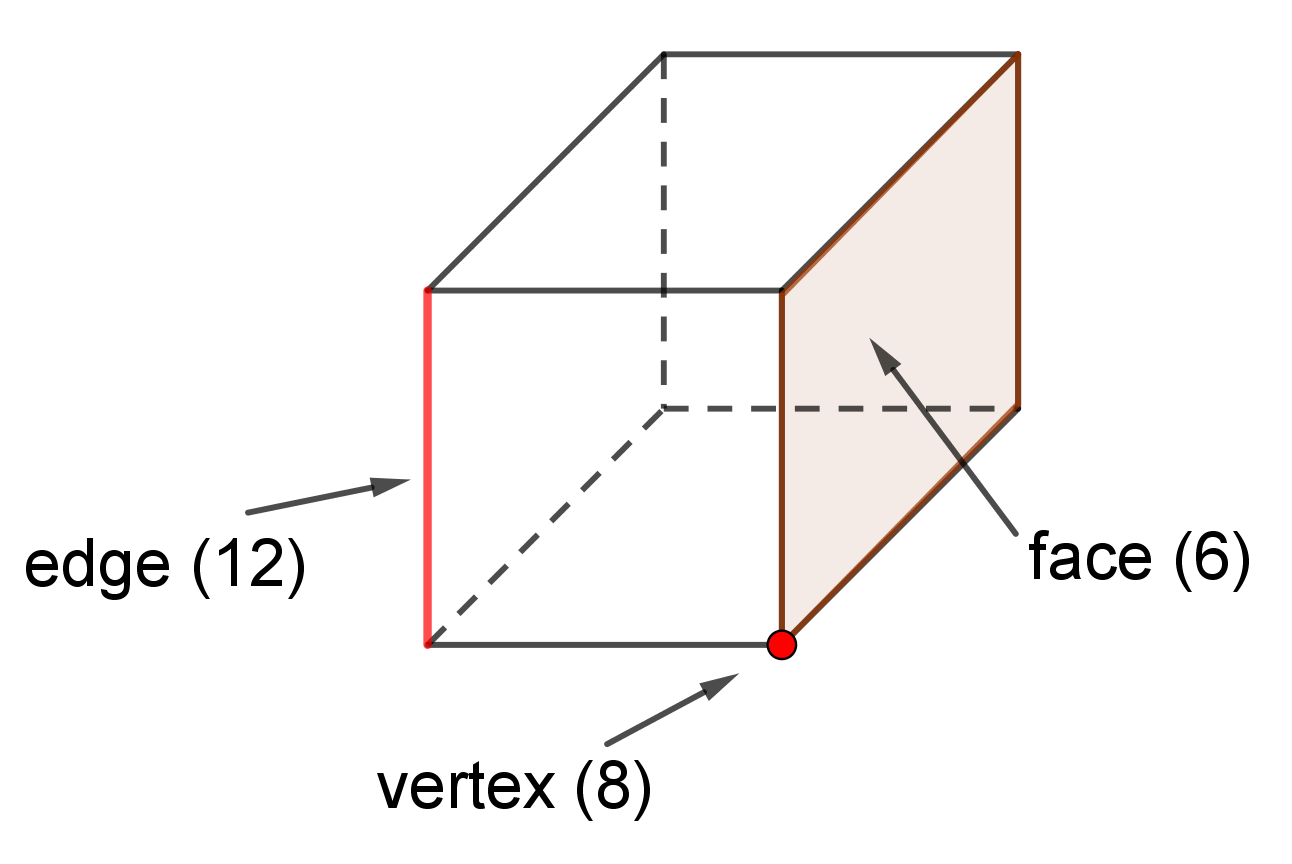If we cut a cardboard box at the edges and put it in a plane, we get a pattern or net for the cube. In general, net can be made for every solid. Net is very useful for finding the surface area of solids. A net for cube is shown below. The surface area of cube is the sum of the areas of each face of the cube. So, surface area of the cube is
$$S=S_1+S_2+S_3+S_4+S_5+S_6$$
Because all faces have equal areas, the surface area is
$$6\times a\times a=6\times a^2$$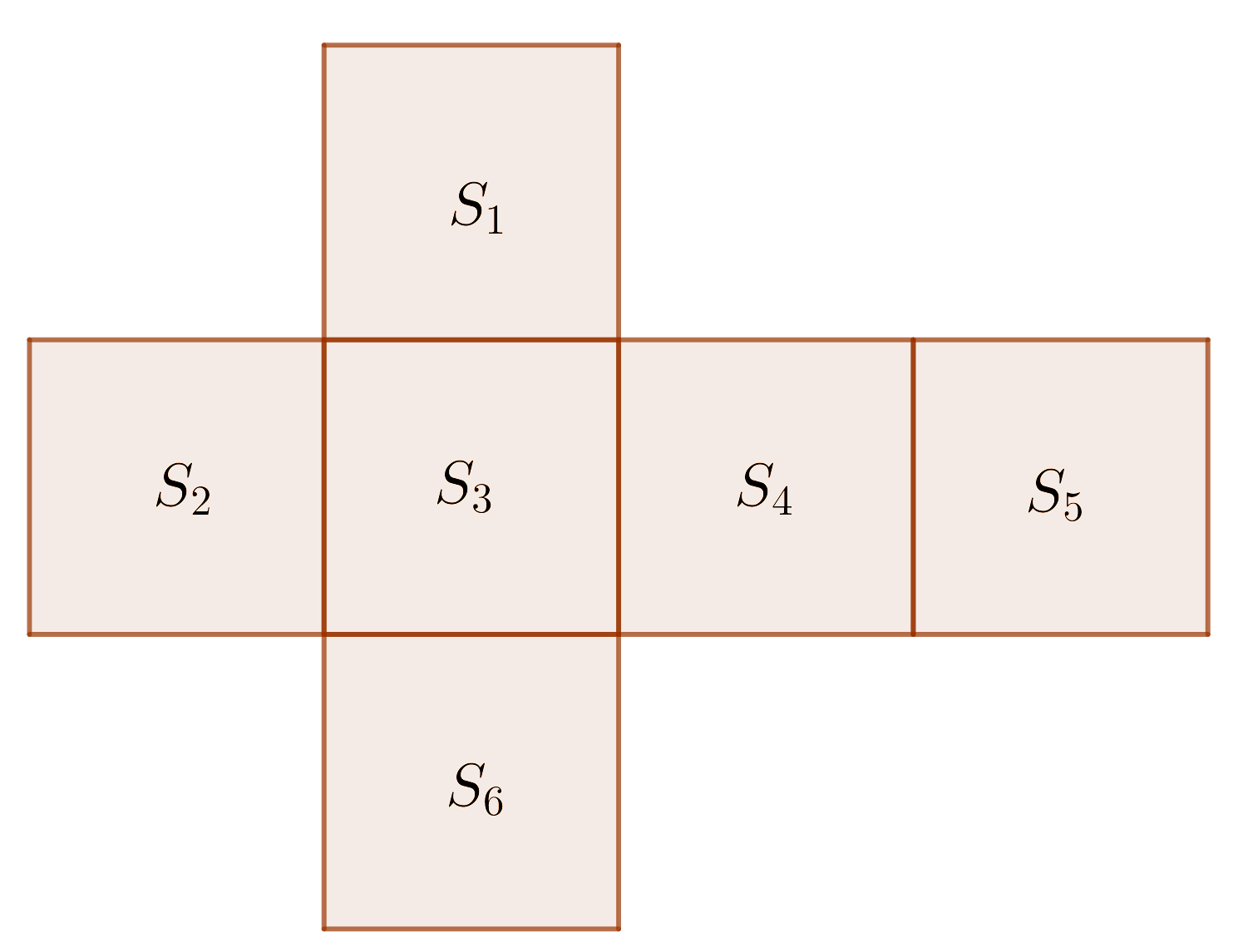The volume of a cube is the measure of the amount of space that a cube contributes. If we multiply the length, width and the height of a cube, we will get the volume formula of a cube. Since the the length, width and the height of a cube are equal to each other, for example $a$, the volume of a cube is
$$V=a\times a\times a$$Doubling the cube, well-known as the Delian problem, is one of the three most famous geometric problems in an ancient period (H. Dorrie, it 100 Great Problems Of Elementary Mathematics, Dover Publications, NY, 1965). Given a cube with the side $a$, find the side of another cube whose volume is twice the volume of the given one. This problem requires the construction of a line segment of length $x$, where $x^3 = 2$, i.e. $x=\sqrt{2}$, the cube root of two. It can be solved by computation but it cannot be solved by a straightedge and compass construction.
The surface area of the cube is measured in units such as square centimeters $(cm^2)$, square meters $(m^2)$, square kilometers $(km^2)$, etc. The volume of a cube is measured in units such as cube centimeters $(cm^3)$, cube meters $(m^3)$, cube kilometers $(km^3)$ etc.
The cube volume and surface area work with steps shows the complete step-by-step calculation for finding the surface area and volume of the cube with the side length of $5\;in$ using the surface area and volume formulas. For any other value for the length of the side of a cube, just supply a positive real number and click on the GENERATE WORK button. The grade school students may use this cube volume and surface area calculator to generate the work, verify the results of the surface area and volume of the three-dimensional bodies or do their homework problems efficiently.

### Real World Problems Using Volume & Surface Area of Cube

Calculating volume and surface area of cube is very important role in mathematics and real life. Ice-cubes, dice, Rubik cube, sugar cubes, gift boxes are some examples of a cube. Many real-life situations can be modeled and analyzed by the surface area and volume of cube. For instance:

• If we want to wrap cube gift box, we should ensure enough wrapper for wrapping;
• If we want to make an aquarium, we should calculate the amount of glass required for making it;
• If we exclude the floor, the cost of painting walls of a cube-shaped room or cube-shaped house can be calculated using the surface area formula;
• If we want to construct a room, then we use the volume of the cube in embroidery designing or pattern designing surface area concept;
• The amount of water formed is equal to the volume of the ice cube, etc.
In arithmetic and algebra, the cube of a number is its third power. This concept can be connected by the volume of cube formula. After cubing any number, we get a number which is called a perfect cube. Cubes of even numbers are always even. Cubes of odd numbers are always odd.

### Cube Practice Problems

Practice Problem 1:
If a cube has a surface area of $864\; cm^2$, find its volume.

Practice Problem 2:
A cube aquarium is $\frac 27$ filled. When we add $16$ liters of water the aquarium is filled $\frac 23$. Find the volume of water that can be found in this aquarium

The cube calculator, formula, example calculation (work with steps), real world problems and practice problems would be very useful for grade school students (K-12 education) to understand the concept of volume & surface area of cube and prism. This concept can be of significance in geometry, to find the volume & surface area of cube and other bodies that can be obtained from two or more cubes. Learning this concept, a student will be able to apply real-life problem solving to analogous mathematical problem solving related to a cube.